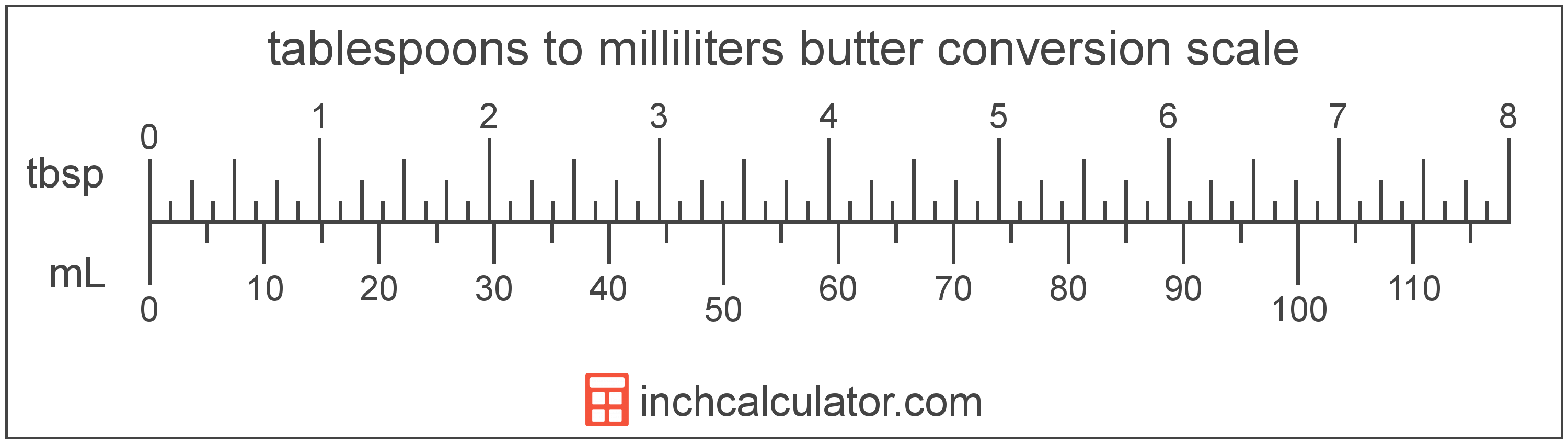# Convert Tablespoons of Butter to Milliliters

Enter the butter in tablespoons below to get the value converted to milliliters.

Results in Milliliters:1 tbsp = 14.786765 mL

Do you want to convert milliliters to tablespoons?

## How to Convert Tablespoons to Milliliters

To convert a measurement in tablespoons to a measurement in milliliters, multiply the butter by the following conversion ratio: 14.786765 milliliters/tablespoon.

Since one tablespoon of butter is equal to 14.786765 milliliters, you can use this simple formula to convert:

milliliters = tablespoons × 14.786765

The butter in milliliters is equal to the butter in tablespoons multiplied by 14.786765.

For example, here's how to convert 5 tablespoons to milliliters using the formula above.
milliliters = (5 tbsp × 14.786765) = 73.933825 mL### How Many Milliliters Are in a Tablespoon of Butter?

There are 14.786765 milliliters in a tablespoon of butter, which is why we use this value in the formula above.

1 tbsp = 14.786765 mL

## What Is a Tablespoon?

One tablespoon of butter is equal to 1/8 of a stick or 1/2 ounce.

The tablespoon is a US customary unit of volume. Tablespoons can be abbreviated as tbsp, and are also sometimes abbreviated as T, Tbls, or Tb. For example, 1 tablespoon can be written as 1 tbsp, 1 T, 1 Tbls, or 1 Tb.

## What Is a Milliliter?

Butter is sometimes measured by volume in milliliters. One stick of butter contains about 118.3 milliliters.

The milliliter is an SI unit of volume in the metric system. A milliliter is sometimes also referred to as a millilitre. Milliliters can be abbreviated as mL; for example, 1 milliliter can be written as 1 mL.

## Tablespoon to Milliliter Conversion Table

Table showing various tablespoon measurements converted to milliliters.
Tablespoons Milliliters
1 tbsp 14.79 mL
2 tbsp 29.57 mL
3 tbsp 44.36 mL
4 tbsp 59.15 mL
5 tbsp 73.93 mL
6 tbsp 88.72 mL
7 tbsp 103.51 mL
8 tbsp 118.29 mL
9 tbsp 133.08 mL
10 tbsp 147.87 mL
11 tbsp 162.65 mL
12 tbsp 177.44 mL
13 tbsp 192.23 mL
14 tbsp 207.01 mL
15 tbsp 221.8 mL
16 tbsp 236.59 mL
17 tbsp 251.38 mL
18 tbsp 266.16 mL
19 tbsp 280.95 mL
20 tbsp 295.74 mL
21 tbsp 310.52 mL
22 tbsp 325.31 mL
23 tbsp 340.1 mL
24 tbsp 354.88 mL
25 tbsp 369.67 mL
26 tbsp 384.46 mL
27 tbsp 399.24 mL
28 tbsp 414.03 mL
29 tbsp 428.82 mL
30 tbsp 443.6 mL
31 tbsp 458.39 mL
32 tbsp 473.18 mL
33 tbsp 487.96 mL
34 tbsp 502.75 mL
35 tbsp 517.54 mL
36 tbsp 532.32 mL
37 tbsp 547.11 mL
38 tbsp 561.9 mL
39 tbsp 576.68 mL
40 tbsp 591.47 mL